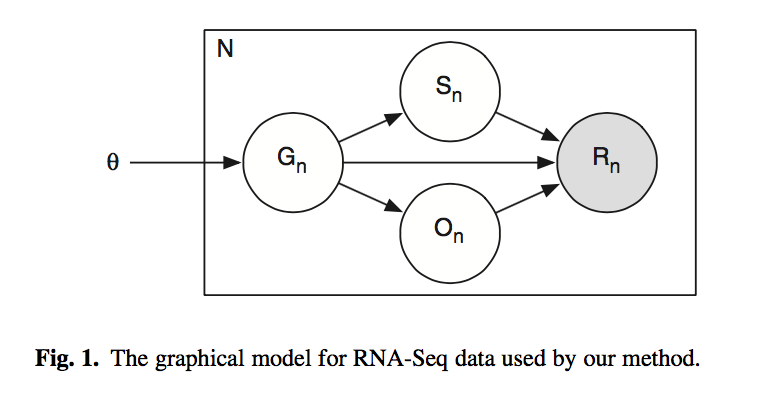# Section 8: Expectation Maximization¶

## Random variables¶

#### Let's start by summarizing what we know about the model we're going to build.¶

We observe the the read sequence:

• $R$ is the observed read sequence ($x_i..x_l$)

We have hidden (or latent) variables that we don't directly observe, but that the model uses to generate the observed data:

• $G$ is the transcript isoform (ranges from $i = 1..M$ with sequence $y_i$)
• $S$ is the start position of the read (ranges from $s = 1..L_i$)

We want to infer the expression level of each transcript:

• $\theta$ is the expression levels, as nucleotide abundances (between 0 and 1)

## Probabilistic graphical models¶

#### Now let's look at the probabilistic graphical model to understand the relationship between our random variables.¶

Probabilistic graphical models (aka Bayesian networks) are directed acyclic graphs (DAGs). In this problem set we know the underlying causal relationships between the variables, so we use a directed graph to represent our model. Probabilistic graphical models allow us to visualize the dependencies of random variables and see which variables are independent of the other variables. This helps us see the assumptions of our model (and as you saw in lecture, it helps us simplify the joint probability calculation!) The shaded variable is our observed data. Our model isn't quite the same as the model below. We have removed the orientation variable.We want to find the probability that the model generates the observed reads $R$, given the nucleotide abundances $\theta$ (this is the likelihood of the model):

$$P(R|\theta)$$

But what we know is:

$$P(R,S,G|\theta)$$

So as we saw in lecture we need to marginalize over the hidden variables in our problem ($S$ and $G$):

$$P(R|\theta) = \sum_S\sum_G P(R,S,G|\theta)$$

First, let's find our joint probability $P(R,S,G|\theta)$:

Using the graphical model above, we can determine the following:

• The probability of selecting isoform $G$ is conditional on $\theta$: $$P(G|\theta)$$
• $S$ and $\theta$ are conditionally independent given $G$: $$P(S|G,\theta) = P(S|G)$$
• $R$ is conditionally independent of $\theta$ given $S$ and $G$: $$P(R|S,G,\theta) = P(R|S,G)$$

Using these independence assumptions, we can factorize and then simplify our joint probability (we do this to get everything in terms of a product of conditional probabilities of individual random variables):

$$P(R,S,G|\theta) = P(R|S,G,\theta)P(S|G,\theta)P(G|\theta)$$$$P(R,S,G|\theta) = P(R|S,G)P(S|G)P(G|\theta)$$

Next, let's write these conditional probabilities in terms of variables we know:

$P(R|S,G)$ is the probability of a read given the segment and gene. We either observe the read sequence at the given position in the transcript ($P(R|S,G) = 1$) or we don't ($P(R|S,G) = 0$):

$$P(R|S,G) = \left\{ \begin{array}{ll} 1 & \text{if } x_1...x_l = y_{i,s}...y_{i,s+l-1} \\ 0 & \text{otherwise} \\ \end{array} \right.$$

$P(S|G)$ is the probability that a segment $S$ is in the given transcript $G$, which is inversely propositional to the length of that transcript:

$$P(S|G) = 1/L_i$$

$P(G|\theta)$ is the probability of a transcript given the parameters, which is equal to the nucleotide abundance of that transcript:

$$P(G|\theta) = \nu_i$$

Finally, let's write our likelihood calculation:

Now our likelihood calculation is:

$$P(R|\theta) = \sum_S\sum_G P(R|S,G)P(S|G)P(G|\theta) = \sum_S\sum_G P(R|S,G)\nu_i/L_i$$

## Generative model¶

#### We can use the model to simulate positive control data that we can later use to test our algorithm.¶

Step 1: select a transcript isoform $G=i$ with probability $\theta_i$

• Don't forget to convert transcript abundances $\tau_i$ to nucleotide abundances $\nu_i$ using the relationship $\nu_i = \frac{\tau_iL_i}{\sum_j{\tau_jL_j}}$

Step 2: select a start position $S=s$ with probability $1/L_i$ (assuming a uniform distribution)

Step 3: generate a read sequence $R = x_1..x_l$, given $G=i$ and $S=s$

## Likelihood of model¶

#### We can calculate the likelihood of of the data given the parameters, which we'll later optimize using EM.¶

As we showed above, the likelihood of the data if the model and its parameters ($\theta$) are known is:

$$P(R|\theta) = \sum_S\sum_G P(R|S,G)P(S|G)P(G|\theta) = \sum_S\sum_G P(R|S,G)\nu_i/L_i$$

## Expectation maximization¶

#### Since we have a "classic chicken and the egg problem," we can use EM to optimize our parameters.¶

We want to find which transcript each of the reads came from. If we knew the counts of reads mapping to each transcript, we could estimate the parameters $\theta$. If we knew $\theta$, we could estimate the counts. But we don't know either one. So we use EM!

• EM finds a local optimum, but Li et al. (2010) show that there is only a single optimum for $\theta$ so we will be finding the global optimum in this case!

For the expectation step, we infer the expected counts $c_i$ mapping to each transcript, given the current parameters $\theta$. This requires calculating the probability that a read mapped to each transcript: $$P(G|R,\theta) = \frac{\sum_S P(R,G,S|\theta)}{\sum_G \sum_S P(R,G,S|\theta)}$$

But we know from our likelihood calculation that $P(R|S,G)\nu_i/L_i$, so:

$$P(G|R,\theta) = \frac{\sum_S P(R|S,G)\nu_i/L_i}{\sum_S\sum_G P(R|S,G)\nu_i/L_i}$$

Now to get the counts assigned to each transcript, we sum over the probabilities for all reads:

$$c_i = \sum_n P(G_n=i|R_n,\theta)$$

For the maximization step, we calculate the updated $\theta$, given the $c_i$ from the previous expectation step. To do this, we simply normalize the counts in order to get nucleotide abundances: $$\theta_i = c_i/\sum_j c_j = c_i/N$$

We continue this iterative process until we have maximized the likelihood of the data given the parameters.

Step 1: initialize $\theta$ to anything (can be random)

• Hint: check out the section in the lecture notes on the Dirichlet distribution

Step 2: (expectation) calculate $c_i = \sum_n P(G_n=i|R_n,\theta)$

Step 3: (maximization) calculate updated $\theta_i = c_i/N$

Step 4: repeat EM until converged

For this problem you should just operate with nucleotide abundances during the EM and then convert to transcript abundances after convergence.